﻿ Numerical Simulation of Cross Flow in In-Line Square Tube Array to Estimate the Convective Heat Transfer CoefficientPublications are Open
Access in this journal
Article Versions
Export Article
• Normal Style
• MLA Style
• APA Style
• Chicago Style
Review Article
Open Access Peer-reviewed

### Numerical Simulation of Cross Flow in In-Line Square Tube Array to Estimate the Convective Heat Transfer Coefficient

Hossin Omar , Suliman Alfarawi, Azeldin El-sawi, Mohammed Zeo
American Journal of Energy Research. 2021, 9(2), 84-91. DOI: 10.12691/ajer-9-2-2
Received September 15, 2021; Revised October 17, 2021; Accepted October 26, 2021

### Abstract

Flow cross tube banks is an important application of different types of heat exchangers, such as compact heat exchangers, and baffled shell and tube heat exchangers. Baffles are used in shell and tube heat exchangers to allow the flow to become cross the tubes. This ensures the mix and increases the convective heat transfer coefficient. Design models of baffled shell and tube heat exchanger, which ensures the cross flow, relay on convective heat transfer coefficient. Which obtained from empirical correlation available in the literature. This work is a numerical approach to simulate flow cross a single tube and an in-line square tube array to estimate the convective heat transfer coefficient. This approach is an alternative to the experimental approach. In order to calculate the heat transfer coefficient and it’s relation to the Reynolds number for a single tube and for an in-line square tube array, a Computation Fluid Dynamic [CFD] software (ANSYS FLUENT), Which utilizes Reynolds Average Navier Stokes [RANS] method to solve the momentum equation in 3-D, was utilized to conduct the numerical simulations. Each model was simulated at 4 different entry velocities of (10, 15, 20, 25 m/s) for a Reynold’s number ranging between 6000-35000. The turbtulence model used was K-ω sst. The results obtained via the CFD simulations were validated with an empirical correlation for the two models. These results have deviations from the empirical results ranging between 5 to 22%. The numerical simulation and the empirical correlation results have identical trends for the case of a single tube and for the case of in-line square tube array. For further improvement in results validations, further studies should be made.

### 1. Introduction

Cross flow over single tubes and tube bank are a classic application for heat transfer, as a matter of fact most heat exchangers are designed based on these fundamental concepts. Recently many studies have been made to examine the flow and heat transfer for different structures of tube banks both in-line and staggered, and their response to changes of flow conditions. Consequently, a large number of experimental and numerical studies have already been carried out to determine heat transfer and flow structures for single tubes and tube banks under different conditions to consider the effects of the Reynold's number, geometry and other several conditions. Focusing mostly on the heat transfer coefficient but also going into the dynamics of the flow, a small discussion about the effect of adding more rows on both pressure drop and the Nusselt number, and how the formation of vortices affects the streamline and what effect does the geometry have on the formation of those vortices. ANSYS Fluent CFD, Fluent is one of the two computational fluid dynamics packages included with the ANSYS computational mechanical software suite. Fluent is a Green-Gauss Finite Volume Method with a Cell-Centered formulation. Computational engineers solve Partial Differential Equations [PDE] using one of the three numerical methods: Essentially, the Finite Difference Method [FDM] discretizes the classical form of the PDE; the Finite Element Method [FEM] discretizes the weak form of the PDE; and the Finite Volume Method [FVM] discretizes the conservative form of the PDE. FVM has the relative advantage of being mathematically straightforward.

In this paper we are studying the effect of changing the value of Reynold’s number on the heat transfer coefficient using Ansys fluent software which is like most fluid simulating software utilizes the power of Finite volume method. Which is a numerical method of solving PDE which are encountered in fluid flow and heat transfer problems, which are called the GOVERNING EQUATIONS which are simplified using the method mentioned. Buyruk et al 1, have made Numerical calculations for laminar flow and heat transfer for a flow past a single cylinder in a row of cylinders. The numerical investigation has considered the effect of blockage and Reynold’s number on the heat transfer and flow characteristics. Kim 2, An analytical study using a CFD (Computational Fluid Dynamics) code has been performed to investigate the effect of the longitudinal pitch on the single-phase heat transfer characteristics in crossflow over inline tube banks. Rehim 3, presented a numerical study of heat transfer and turbulent fluid flow over a staggered circular tube bank using a CFD software (Ansys fluent). The model for staggered arrangements is applicable for use over a wide range of the aspect ratio 𝑆𝑇 𝑆𝐿 ⁄ when determining heat transfer and turbulent fluid flow over a staggered circular tube bank. Nabeel Abeda and Imran Afgan 4, reported the effect of changing the aspect ratio on the heat transfer and flow quantities over in-line tube banks. Two types of in-line arrangement were employed, square and non-square configurations. The models that were examined are a standard k-ε model, SST k-ω model, v2-f model, EB k-ε model and EB-RSM model. Bender et al 5, this work presents a numerical study addressing the hydrodynamic and thermal behavior of a new trapezoidal tube bank arrangement composed of nine circular cylinders subjected to forced convection. The pressure drop and Nusselt numbers are evaluated aiming to assess the influences of the following parameters: the new trapezoidal factor, the longitudinal and transverse pitches and the free stream velocity (Reynold’s number). Peter D Souza el al 6, represents a two dimensional numerical study of the performance of an evaporator of a window type air conditioner. The analysis of the performance of the heat exchanger is done using the software ANSYS CFD with finite volume discretization. So, in this paper we are going to utilize a fluid simulation software (Ansys fluent) and validate our results using experimental equation (empirical correlation) 7, 8.

This work aims to numerical simulate the cross flow in single tube and in inline square tube bank utilizing the commercial CFD software (ANDYS-FLUENT). with a simple scheme with second order upwind for the conservation equation and for the turbulence and using second order equations for solving the pressure distribution. Using SST k-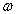as the turbulence models for both the single tube and for in-line square tube bank in cross flow. Simulations will be conducted for different Reynolds number and estimating the average convective heat transfer coefficient at each Reynolds number. Estimated convective heat transfer coefficient (h) will be validated with results calculated via empirical correlations 7, 8 for single tube and inline tube bank in cross flow. More simulations could be conducted using other turbulence models such as (k-, k-ε) to investigate the effect of turbulence models on the resultant convective heat transfer coefficient. Mesh sensitivity study will be performed to find the optimum number of nodes to increase the accuracy of resultant convective heat transfer coefficient.

### 2. Governing Equations

The governing equations of a mathematical model describe how the values of the unknown variables (i.e. the dependent variables) change when one or more of the known (i.e. independent) variables change. The governing equations of fluid flow represent mathematical statements of the conservation laws of physics.

2.1. The Mass Conservation in Three Dimensions

Mass conservation equations (or continuity equations) simply relate the mass rate change in a. unit volume with the sum of all the species entering/exiting this volume at a given period is equal to Zero. The general equation for mass conservation, for an steady, three-dimensional mass conservation or continuity equation, is given by:(1)
2.2. Momentum Equation in Three Dimensions

Newton’s second law states that the change rate of momentum of a fluid particle equals the sum of the forces on the particle, the x-component of the momentum equation is found by setting the rate of change of x-momentum of the fluid particle equal to the force in the x-direction on the element due to the surface stresses plus the rate of increase of x-momentum due to sources:

Conservation of Momentum Equations:

X-momentum: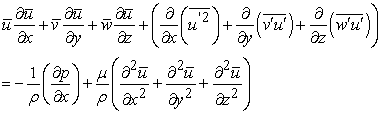(2)

Y-momentum:(3)

Z-momentum:(4)
2.3. Energy Equation in Three Dimensions

The energy equation is derived from the first law of thermodynamics which states that the rate of change of energy of a fluid particle is equal to the rate of heat addition to the fluid particle plus the rate of work done on the particle.

Conservation of Energy Equation: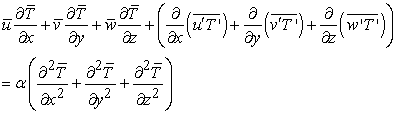(5)

Turbulence Kinetic Energy Equation: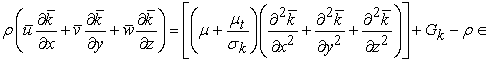(6)

### 3. Problem Definition

The non-dimensional factors applied here such as, Nusselt number (Nud), Reynold’s number (Red) and are expressed as a Reynold’s number flow past a single tube:(7)

The average Nusselt number for a single tube an empirical correlation from Hilbert: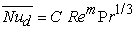(8)

Reynold’s number flow past a tube bundle:(9)

For the in-line arrangement, Vmax occurs at the transverse plane A1 of Figure 1a, and from the mass conservation requirement for an incompressible fluid 7, 8:(10)

It will occur at A2 if the rows are spaced such that 7, 8:(11)

Since our model doesn’t satisfy the previous equation the maximum speed will occur at A1.

The average Nusselt number for a tube bundles (both inline and staggered) 7, 8.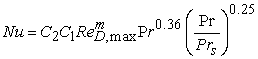(12)

where C1 and C2 are constants 7, 8.

• Figure 1. Tube arrangements in a bank. (a) Aligned. (b) Staggered [7,8]

### 4. Model Description

Two models (single tube and in0line square tube array) were made using ANSYS design modeler software. For both models , a 200 mm entry section was added to ensure a fully developed velocity profile to maintain a steady analysis, and a 600 mm section after the tubes was also added to study the flow in that region and its effect on the results For single tube model, as shown in Figure 2 a tube diameter of 15 mm was used.

In-line square model as shown in Figure 3, consists of five columns and nine rows down stream with a tube diameter of 15 mm and4.1. Mesh Structure

A multi-zone method with hexa-mesh structure was used with adding inflation layers on the tube surfaces in order to maintain high density mesh where there are significant changes.

The Figure 4, Figure 5 display the mesh structure for the single tube and for an in-line square tube models, noticing that high density mesh is closer to the tube surface and a slightly coarser mesh as we go further away from it.

• Figure 2. 3-dimensional model designed using Ansys Design modeler, displaying the model used for simulating the flow across a single tube
• Figure 3. 3-D model displaying the model used for the flow across an inline tube bank
• Figure 4. 2-Dimentional view of the mesh sizing/distribution used to run the simulation for the flow across a single tube. And the mesh was done using ANSYS Meshing
• Figure 5. The mesh sizing and distributing on the in-line square tube bank model

### 5. Results and Discussion

The calculation for both the single tube and the tube bank were done at almost the same settings and but never the less, every detail about the setting will be mentioned on every section.

5.1. Heat Transfer Coefficient

Both the empirical calculations results and the computational results are given in this section in both tabular and in form of charts. A free stream of air cross flowing over a single tube and over the in-line square tube bank where the free stream temperature is 15°C and the pipe is at 150°C and the free stream velocity is entering at four different Velocities of (10, 15, 20, 25) m/s, using the equations mentioned in the previous section and using the CFD software we obtained the following results: in case of single tube model, as shown in Table 1 and in Figure 6, the values of the heat transfer coefficient diverges as the value of the Reynolds number increases, the deviation between the numerical and empirical results has a value of 4% at Reynolds of 6800 and it reaches to a value of about 14.5% at Reynolds number of 17000. In case of in-line square tube array, as shown in Table 2 and in Figure 7, the values of the heat transfer coefficient starts to converge as the value of the Reynolds increases for the in-line square tube array. And the deviation between the empirical and numerical simulation results has higher of 22% at lower end Reynolds number and it decreases to a value of 13.7% at the higher end Reynolds number.

• Figure 6. Displaying Reynolds vs. both empirical and numerical values of the heat transfer coefficient for single tube
• Figure 7. Displaying Reynolds vs. both empirical and Numerical values of the heat transfer
5.1. Temperature Contours

In this section, temperature contours for single tube and for in-line square tube models are displayed using Ansys post processer at the free stream velocity of 25m/s, as shown in Figure 8, Figure 9 respectively. Fluid temperature has higher values closer to the tube wall for both cases. For a single tube, as shown in Figure 8, the region behind the tube has higher temperature than the upstream region.

In case of in-line square model as shown in Figure 9, which has similar behave as the case of the single model, also it the gap between tubes temperatures increases down stream as show in Figure 9.

5.3. Pressure Contour

In this section, we will be displaying the pressure contour for the single tube, and for in-line square tube bank. For a single tube as shown in Figure 10, the pressure is highest at the stagnation point upstream of the tube and it decreases down stream of the tube and it is has the minimum values starting from the separation points of flow and behind the tube. In case of in-line square tube array arrangement as shown in Figure 11, the pressure has the highest values at the stagnation points of the first tube and their decreases down stream and the minimum values occurs starting from yj seventh row. Which refers to the pressure drop corresponding with the flow.

• Figure 8. The temperature contour for a cross flow long a single tube at a free stream velocity of 25 m/s
• Figure 9. The temperature contour for a cross flow long a staggered tube bank arrangement at a free stream velocity of 25 m/s
• Figure 10. Pressure contour for a cross flow long a single tube at a free stream velocity of 25 m/s
• Figure 11. Pressure contour for a cross flow long an inline tube bank arrangement at a free stream velocity of 25 m/s
• Figure 12. Velocity streamlines for a cross flow long a single tube at a free stream velocity of 25 m/s
• Figure 13. Velocity streamlines for a cross flow long an inline tube bank arrangement at a free stream velocity of 25 m/s. Showing a maximum velocity of about 63m/s
5.4. Flow Structure and Velocity Streamlines

The flow structure of a single tube and for in-line square tube array are presented in Figure 12 and Figure 13 respectively. In case of a single tube as shown in Figure 12, the flow velocity has a low value at a stagnation point and the stream lines separate at approximately 10° and stream lines circulate behind the tube and forms vortices downstream. The flow velocity gas the highest values in the upper and down regions of the tube, which is the gap between tube walls and the upper and down walls of the duct.

In the case of in-line square tube array, as shown in Figure 13, the stream lines separate at an approximately 10° and then it circulates forming vortices behind each of tube. While the flow velocity has its highest values in the gap between tube columns.

### 6. Conclusion

Numerical simulation results in case of a single tube are more accurate than those in case of in-line square tube array. The deviations between CFD simulation and empirical calculations in case of a single tube at a low Reynolds number is 4%, while it increases with increasing Reynolds number and reaches to 14.56% at a high Reynolds number, which means the two approaches diverge with increasing of Reynolds number. While in the case of in-line square tube array, the results of both approaches; CFD and empirical; diverge with increasing of Reynolds number. Numerical simulation is a viable approach to simulate cross flow in tube arrays to estimate the convective heat transfer coefficient. However, more improvement in CFD results could be obtained by increasing of number of nodes in the model by conducting mesh sensitivity study, which we are currently doing.

### Nomenclature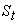transverse pitch [m].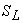longitudinal pitch [m].Density of the fluid [kg/m3 ].

D tube outside diameter [m]Free stream Velocity [C]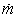Mass rate of flow of either the hot or cold streams. [Kg/s]heat transfer coefficient using empirical formula [W/m2.C]heat transfer coefficient using Ansys fluent [W/m2.C].

k Thermal conductivity of theDiagonal pitch [m]longitudinal pitch [m].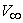Upstream Fluid velocity [m/s]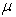Viscosity if the fluid [N.s/m2]

### References

  E. Buyruk, M.W. Johnson, I. Owen, Numerical and experimental study of flow and heat transfer around a tube in cross-flow at low Reynolds number, Int. J. Heat Fluid Flow. 19 (1998). In article View Article  T. Kim, Effect of longitudinal pitch on convective heat transfer in crossflow over in-line tube banks, Ann. Nucl. Energy. 57 (2013) 209-215. In article View Article  Z. S. Abdel-Rehim, Heat Transfer and Turbulent Fluid Flow Over a Staggered Circular Tube Bank. Mechanical Engineering Department, National Research Center, Dokki, Giza, Egypt, 2014. In article View Article  Nabeel Abeda and Imran Afgan, A CFD study of flow quantities and heat transfer by changing a vertical to diameter ratio and horizontal to diameter ratio in inline tube banks using URANS turbulence models. Institute of Anbar, Middle Technical University, Iraq (2017). In article View Article  A. Bender, A.M. Meier, M. Vaz, P.S.B. Zdanski, A numerical study of forced convection in a new trapezoidal tube bank arrangement, Int. Commun. Heat Mass Transf. 91 (2018) 117-124. In article View Article  Peter D Souza, Deepankar Biswas, Suresh. Deshmukh, Air side performance of tube bank of an evaporator in a window airconditioner by CFD simulation with different circular tubes with uniform transverse pitch variation, Department of Mechanical Engineering, Veermata Jijabai Technological Institute, Mumbai, India 2020. In article View Article  Bergman, T., Lavine, A., Incropera, F. and Dewitt, D. (2011). Fundamentals of Heat and Mass Transfer. 7th Edition, John Wiley and Sons, Jefferson City. In article  A. Zukauskas, Heat transfer from tubes in crossflow, Adv. Heat Transf. 18 (1987) 87-159. In article View Article  H. Versteeg, (1996), An Introduction to Computational Fluid Dynamics: The Finite Volume Method Approach, Longman House, Burnt Mill, Harlow. In article  Zena K. Kadhim, Muna S. Kassim, Adel Y. Abdul Hassan, Effect of Integral Finned Tube on Heat Transfer Characteristics for Cross Flow Heat Exchanger, International Journal of Computer Applications, Volume 139 – No.3, April 2016. In article View Article  Priyanka G, M. R. Nagraj, CFD Analysis of Shell and Tube Heat Exchanger With and Without Fins, International Journal of Science and Research, (2012). In article

Published with license by Science and Education Publishing, Copyright © 2021 Hossin Omar, Suliman Alfarawi, Azeldin El-sawi and Mohammed ZeoThis work is licensed under a Creative Commons Attribution 4.0 International License. To view a copy of this license, visit http://creativecommons.org/licenses/by/4.0/